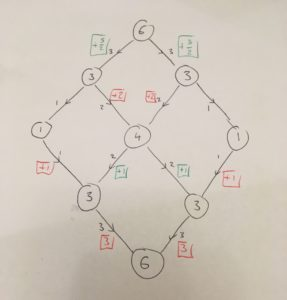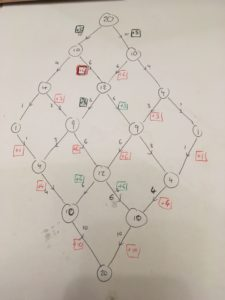“That looks straightforward,” I thought. “I’ll keep on looking at this geometry puzzle.” Nut-uh.

A standard pack of 52 cards is shuffled. The cards are turned over one at a time, and you guess whether each will be red or black. How many correct guesses do you expect to make?

I’m going to present a neatly tidied-up answer after the line, but it’s worth mentioning that this was far from the first method I came up with.

### Heuristics

I think a lower bound is 26, because whenever there is an imbalance in the reds and blacks, which is at least half the time, you have a better than 50-50 chance of picking correctly.

As for an upper bound, I would reason that the more cards you have, the smaller your percentage correct will be, and if you have two cards, you would expect 75% - so an upper limit would be 39 cards. Where did the 75% come from? Read on.

### Paths on a lattice: 2 cards

Two big elements of my problem-solving practice are:

• Having a good diagram; and
• Reducing the size of the problem

So, I drew out what started as a probability tree for the two-card situation:The two-card lattice

Only it became a lattice. Assuming I choose randomly when I have an equal number of cards left, I get half a point (on average) from my initial guess, and then I know what colour the remaining card is and get a point for that. I score 1.5 points for each path, which is my expected score.

### Paths on a lattice: 4 cards

With four cards, the lattice becomes more complicated: there are $\nCr{4}{2} = 6$ paths through the grid, and I think it makes sense to count how many go through each point.

The six paths split evenly between the two following nodes, in each case leaving one card of one colour and two of the other. So a third of the time, you’ll guess wrong and go to a 2-0 state, and two-thirds of the time you’ll come back to the two-card case.

And here we start to notice something: every time you move towards the central column of nodes, you get a point. When you move away from it, you get nothing - except when you’re moving directly off of the column, in which case you get half a point.

Adding all of this up gives 17 points from six paths, or an expected score of just under 3.The 4-card lattice

And that leads to a further observation: every path through the lattice must move towards the diagonal twice and away twice. (In general, with $2n$ cards, you move towards the diagonal $n$ times and away $n$ times.)

This suggests a form for the answer of $n + \frac{1}{2}D(n)$, where $D(n)$ is the number of times you expect a path to reach the diagonal.

### Paths on a lattice: 6 cards

Let’s check it with a 6-card set-up: there are 20 paths, and I expect the total number of points to be $20 \times 3 + \frac{1} {2}d(3)$, where $d(3)$ is the total of paths on the central column (except the last one). I’ve used $d$ for the total number of paths, as opposed to $D$ for the expected number.

Drawing it out, I can see that there are $64-20 = 44$ visits to the diagonal, so there should be $60 + 22 = 82$ points altogether - which matches the total in the red boxes!The 6-card lattice

### What’s the pattern?

In the two-card case, there were four visits to the diagonal (although we ignored the concluding two).

In the four-card case, there were sixteen, and we ignored the final six.

With six cards, there were 64, and we cast out 20.

It looks very much like the number of diagonal visits for the $2n$-card case is $2^{2n}$!

(In fact, it is - but I have only proved it by looking it up on OEIS rather than doing sums. There’s a challenge for you!)

This tells us that $d(n) = 2^{2n} - \nCr{2n}{n}$.

### Putting it all together

In the $2n$-card case, there are $\nCr{2n}{n}$ paths, which between them score $\left(\nCr{2n}{n}\right)n + \frac{1}{2}\left(2^{2n} - \nCr{2n}{n}\right)$ points. So, the expected number of points is simply $n + \frac{1}{2}\left(\frac{2^{2n}}{\nCr{2n}{n}} - 1\right)$

In the case where $n=26$, we get a total of $26 + \frac{1}{2}\left(\frac{2^{52}}{\nCr{52}{26}} - 1\right)$, which is…

#### Whoosh

I might have known to expect you, sensei! Is that a dagger made of silver?

Sterling silver.”

Seems impractical. Oh wait! Is that a hint? We could use Stirling’s approximation to make that nicer, couldn’t we? Spelt differently, though. But… yes, you’re absolutely right, just move the dagger away from my neck and nobody gets hurt. Especially not me.

OK, so Stirling says $n! \approx \sqrt{2\pi n} \left(\frac{n}{e}\right)^n$. So, $\nCr{2n}{n}$, for large enough $n$, is $\frac{ 2 \sqrt{\pi n} \left(\frac{2n}{e}\right)^{2n}}{2\pi n \left(\frac{n}{e}\right)^{2n}}$. Surely you don’t expect me to simplify that in my head?

Don’t raise your eyebrow like that.

Fine. Well, we’ve got $\frac{1}{\sqrt{n\pi}}$ from the pi-ey bits, and… $2^{2n}$ from the brackets? Looks ok. So $\nCr{2n}{n} \approx \frac{2^{2n}}{\sqrt{n\pi}}$.

And since we want $2^{2n}$ divided by that, we just get $\sqrt{n\pi}$!

“Which, for $n=26$, is 9.08. I won’t bore you with the details.”

Then subtract one and halve to get 4.04, making 30.04 as the expected score!

• Thanks to @Barney_MT and the @EastDorMathsJam< crowd for their input!